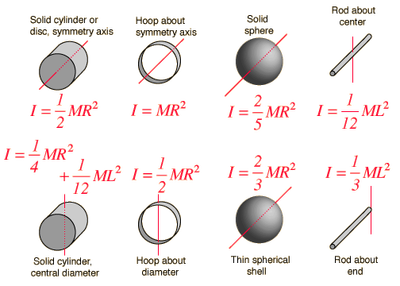## FANDOM

19,867 PagesThe formula of the rotational energy is K= 1/2* Ι*ω^2

The moment of inertia of a sphere is 2/5mR^2

The Earth's angular velocity is 7.3*10^-5 rad/s

Earth's Mass = 5.97e24 kg

Earth's radius = 6372000 m

Κ = 1/2*Ι*ω^2 = 1/5 * m*R^2 *ω^2 = 2.58e29 Joules (Moon level)